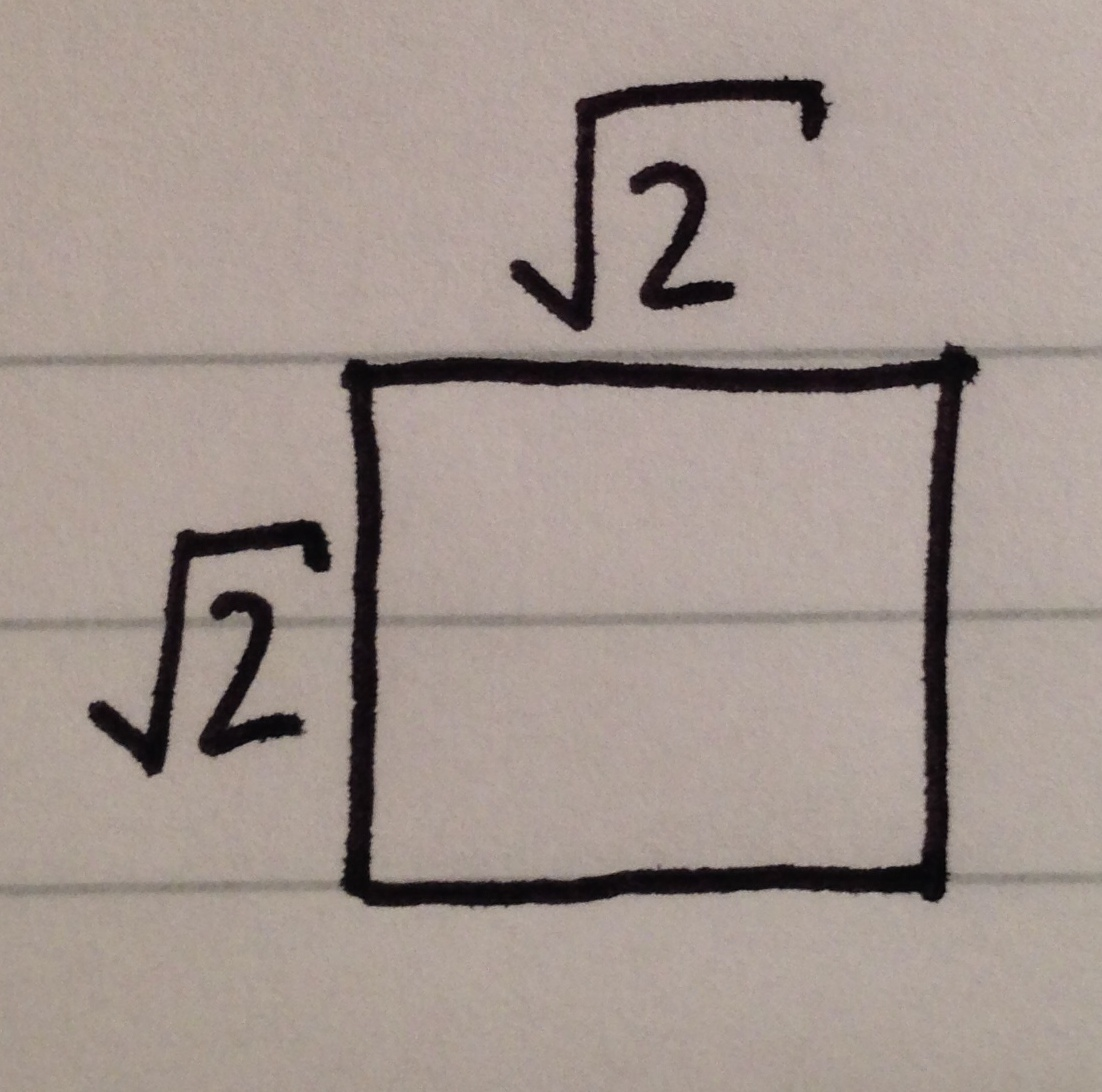# The Mathematicians Square[Root]

Geometry Level 1A Mathematician has a square of sides length $\sqrt { 2 }$

He's very clever and is able to work out the area of the square.

However, he suddenly sees that he can write his answer for the area out in a different way! In the form of:

$\sqrt { x\sqrt { x\sqrt { x\sqrt { x\sqrt { x.... } } } } }$

What is the value of x?

×

Problem Loading...

Note Loading...

Set Loading...